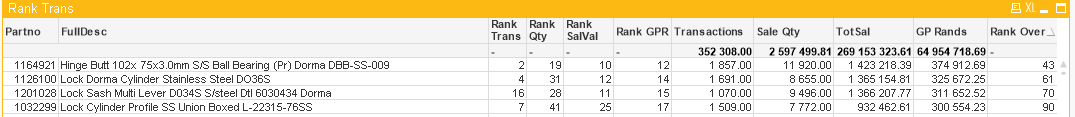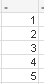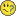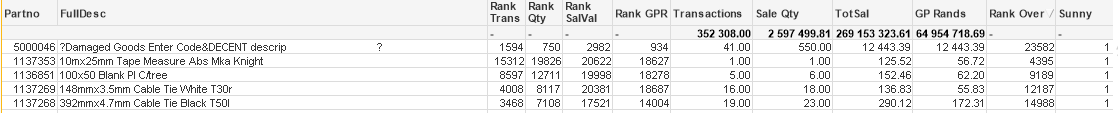# QlikView App Dev

Discussion Board for collaboration related to QlikView App Development.

Announcements
cancel
Showing results for
Did you mean:Contributor III

## Find the rank of multiple ranked fields

Morning to all,

Could I please get some help with the following.

I have 4 unique ranking calculation which give me the respective rank based on

• Qty of Tansactions (Rank Trans)
• `only(aggr(nodistinct rank(count(Partno),4,1), Partno))`
• Qty Sold (Rank Qty)
• ```only(aggr(nodistinct rank(sum(OrderQty),4,1), Partno))
```
• Sales Value (Rank SalVal)
• ```only(aggr(nodistinct rank(sum(TotalSales),4,1), Partno))
```
• GP Rands (Rank GPR)
• ```only(aggr(nodistinct rank(sum(RProfit),4,1), Partno))
```All of which are working perfectly.

What I require is to get an overall ranking based on all of the previous rankings. (Rank Over)

Where currently I can only get the combined sum of the rank.

ie in the Rank Over the results should display :1 Solution

Accepted SolutionsMVP

Try this

Rank(Total(-RangeSum(

only(aggr(nodistinct rank(count(Partno),4,1), Partno)),

only(aggr(nodistinct rank(sum(OrderQty),4,1), Partno)),

only(aggr(nodistinct rank(sum(TotalSales),4,1), Partno)),

only(aggr(nodistinct rank(sum(RProfit),4,1), Partno)))),4,1)

7 RepliesMVP

Rank(Expression1, Expression2, Expression3, Expression4)Contributor III
Author

I have thanks, as well as adding the four ranks together and then doing an overall ranking on that result.MVP

Sorry, that is what I meant

Rank(RangeSum(

only(aggr(nodistinct rank(count(Partno),4,1), Partno)),

only(aggr(nodistinct rank(sum(OrderQty),4,1), Partno)),
only(aggr(nodistinct rank(sum(TotalSales),4,1), Partno)),

only(aggr(nodistinct rank(sum(RProfit),4,1), Partno))))

What do you do when you add this as one of your expressionContributor III
Author

Ok no laughing now,I modified your suggestion to get the ranking to work. (Rank Over)

Rank(Total(RangeSum(

only(aggr(nodistinct rank(count(Partno),4,1), Partno)),

only(aggr(nodistinct rank(sum(OrderQty),4,1), Partno)),

only(aggr(nodistinct rank(sum(TotalSales),4,1), Partno)),

only(aggr(nodistinct rank(sum(RProfit),4,1), Partno)))),4,1)

And it works perfectly except for one small problem.

It is ranking in the reverse order ????When I use the example you have posted I get (Sunny)@

###### Re: Find the rank of multiple ranked fieldsThanks for the patienceMVP

Try this

Rank(Total(-RangeSum(

only(aggr(nodistinct rank(count(Partno),4,1), Partno)),

only(aggr(nodistinct rank(sum(OrderQty),4,1), Partno)),

only(aggr(nodistinct rank(sum(TotalSales),4,1), Partno)),

only(aggr(nodistinct rank(sum(RProfit),4,1), Partno)))),4,1)MVP
 Ok no laughing now,

Why not? Laughing is a good thingContributor III
Author

You are a star

###### Re: Find the rank of multiple ranked fieldsAppreciate all the help with this.